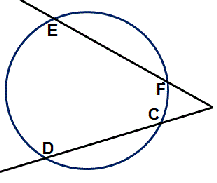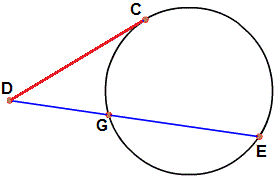﻿ Analytic geometry Circle Formulas
﻿

## Equation of a circle:

In an x−y coordinate system, the circle with center (a,b) and radius r is the set of all points (x,y) such that: $$(x-a)^2 + (y-b)^2 =r^2$$ Circle centered at the origin: $$x^2 + y^2 = r^2$$ Parametric equations \begin{aligned} x &= a + r\,\cos t \\ y&= b + r\,\sin t \end{aligned} where t is a parametric variable. In polar coordinates the equation of a circle is: $$r^2 - 2\cdot r \cdot r_0\cdot cos(\Theta - \phi ) + r_0^2 = a^2$$

## Area of a circle:

$$A = r^2\pi$$

## Circumference of a circle:

$$C = \pi \cdot d = 2\cdot \pi \cdot r$$

## Theorems:

(Chord theorem) The chord theorem states that if two chords, CD and EF, intersect at G, then: $$CD \cdot DG = EG \cdot FG$$(Tangent-secant theorem) If a tangent from an external point D meets the circle at C and a secant from the external point D meets the circle at G and E respectively, then $$DC^2 = DG \cdot DE$$(Secant - secant theorem) If two secants, DG and DE, also cut the circle at H and F respectively, then: $$DH \cdot DG = DF \cdot DE$$ (Tangent chord property) The angle between a tangent and chord is equal to the subtended angle on the opposite side of the chord.